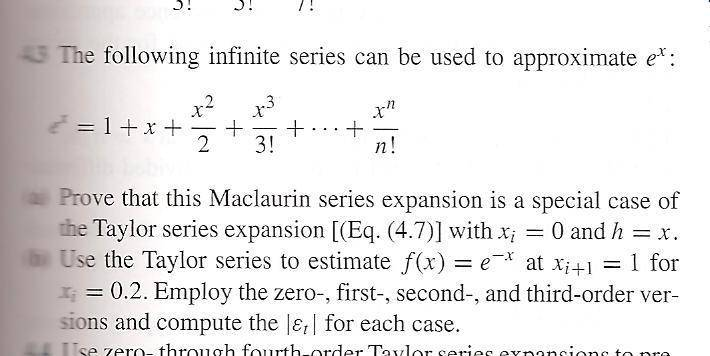# Computing true percent relative error (Taylor Series)

## Homework Statement

See figure attached,## The Attempt at a Solution

Isn't the Maclaurin series just simply the Taylor series around 0?

$$(\text{i.e. } (x-c), \quad c=0, \quad x )$$

Also for part B, how do we go about solving for $$| \epsilon_{t} |$$?

Thanks again!